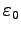## 1. 1. 10 Physical Interpretation

After the mathematical solution is obtained and proven or assumed to be adequate for the posed problem, the mathematical solution may be interpreted in a physical manner. Here, all purely mathematical values gain a physical meaning. As an example, vector functions or vector fields  may be interpreted as velocity fields. The physical solution can be compared to an experiment which is carried out, e.g. to verify or refine the simulation.

Mathematical solutions are typically free of physical units and perhaps scaled with respect to a given system of units, for instance the SI. Constants which occur in physical problems have to be scaled to a special system of units, e.g. the vacuum dielectricity constanthas to be scaled with respect to the scaling of the occurring voltages, distances, time steps, and currents.

For this reason, simulations results where no such special constants occur explicitly, e.g. the Laplace equation (in its mathematical interpretation), may be valid for various simulation problems. However, it has to be remarked that derived quantities, e.g. field strength, depend on the scaling used. The physical interpretation can result in scaling the complete simulation result due to a re-scaling of all stored values.

Michael 2008-01-16